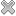Courses / Module

Toggle PrintLINEAR MODELS (R)

Module code: ST833
Credits: 5
Semester: 1
Department: MATHEMATICS AND STATISTICS
International:Overview

Overview
--------
Scatterplots and regression. The multiple regression model and its applications. Matrix notation, mean vectors and covariance matrices. Least-squares estimation. The multivariate normal distribution. Hypothesis testing and confidence intervals, prediction. Simple linear regression as a special case. Analysis of variance, R2, sequential sums of squares, general F-tests. Added variable plots. Model checking via testing for lack of fit and residual plots. Regression diagnostics: residuals, leverage, outliers, influence and Cook's Distance. Polynomials and factors in regression. Weighted least squares.

Lectures and tutorials as for ST303.

For Registered Research students. Permission is required from the Head of the Mathematics & Statistics Department.Learning OutcomesTeaching & Learning methodsAssessmentRepeat optionsPre-RequisitesTimetable